# How do you write the determinant of a matrix in LaTeX?

If A is a matrix, then the matrix determinant is denoted by the following three expressions.Latex has \det command for det(A) expression. Writing direct det in math mode will change the font of the expression which is not the right approach.

\documentclass{article}
\begin{document}
$\det(A)$
$\det A$
% This is not best practis
$det A$
\end{document}

Output :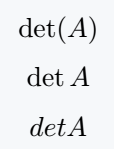However, if you type det in the \mathrm command, the font will not change. There may be a problem with light spaces between expressions and matrix names, which can be fixed using \,.

\documentclass{article}
\begin{document}
% This is not best practis
$det A$
% But use \mathrm command
$\mathrm{det}A$
% Use \, for space
$\mathrm{det}\,A$
$\det A$
\end{document}

Output :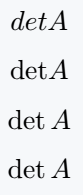All such functions or expressions in mathematics are defined by \mathrm{function}\, syntax in the latex library. Then you can use Direct as a command.

There are multiple commands for the absolute value symbol. Of course, you can use the single bar of your keyboard.

In the following output, you can use what seems to be your best practice, and the only difference is in the space.

\documentclass{article}
\usepackage{amsmath}
\begin{document}
$|A| = \det A$
$\mid A \mid = \mid B \mid$
$\lvert A \rvert = -\lvert C \lvert$
\end{document}

Output :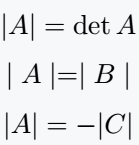In the case of matrix determinants, the elements of the matrix will be bound by a single vertical bar.

For this, you need to use the vmatrix argument in the matrix environment.

\documentclass{article}
\usepackage{amsmath}
\begin{document}
$\det\begin{pmatrix} a_{11} & a_{12} & a_{13}\\ a_{21} & a_{22} & a_{23}\\ a_{31} & a_{32} & a_{33} \end{pmatrix} = \begin{vmatrix} a_{11} & a_{12} & a_{13}\\ a_{21} & a_{22} & a_{23}\\ a_{31} & a_{32} & a_{33} \end{vmatrix}$

$= a_{11}\begin{vmatrix} a_{22} & a_{23} \\ a_{32} & a_{33} \end{vmatrix} + a_{12}\begin{vmatrix} a_{23} & a_{21} \\ a_{33} & a_{31} \end{vmatrix} + a_{13}\begin{vmatrix} a_{21} & a_{22} \\ a_{31} & a_{32} \end{vmatrix}$
\end{document}

Output :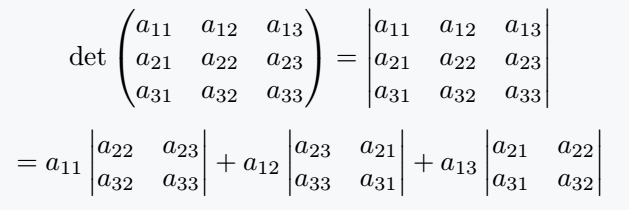And for this environment above, you must import the amsmath package, because there is no default presence in latex.

You will hardly be familiar with the physics package! In this case, I would recommend using the physics package.

Because without using the environment you can use the matrix elements in the predefined \mdet command of this package.

\documentclass{article}
\usepackage{physics}
\begin{document}
$\det X = \mdet{a & b & c\\ d & e & f \\ g & h & i}$
$\det\mqty(\dmat{1,1,1}) = 1$
$\mdet{ A & B \\ C & D } = \det(AD-BC)$
\end{document}

Output :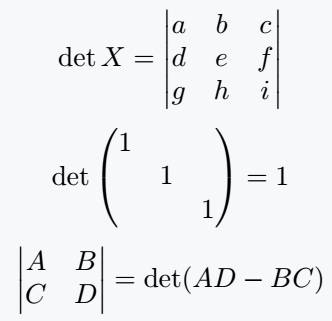#### Md Jidan Mondal

LaTeX expert with over 10 years of experience in document preparation and typesetting. Specializes in creating professional documents, reports, and presentations using LaTeX.